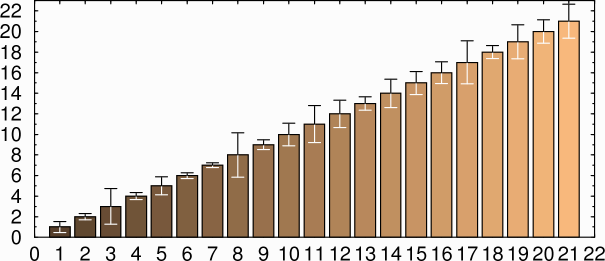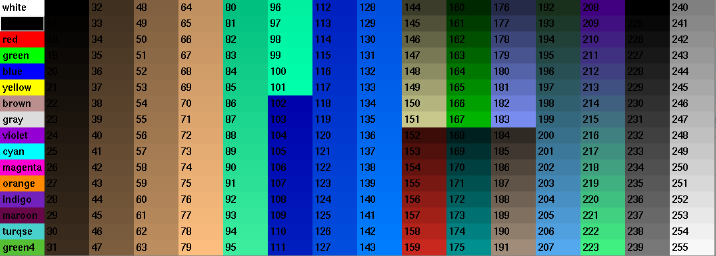Linux Setup Notesnov 12, 2012; last updated may 22, 2018

### Improvements and Bugfixes and for xmgr

Xmgr, the standard Linux graph-drawing program, is no longer being maintained, having been replaced by xmgrace. However, the user interface in xmgrace is a challenge to navigate (see here for the contortions I needed to go through to draw a bar graph). So we would like to keep xmgr running as long as possible. As time goes by, the compiler keeps changing, which causes xmgr to crash. Below are the fixes I've found. Also included is a patch to draw bar charts where each bar is a different color. These fixes apply to version 4.1.2. A patched version is available here.

On my computer, xmgr crashes at startup before it even puts up a window. The problem is in line 1295 in motifutils.c, where it creates a Motif cascaded menu:

```cascadeTmp = XtVaCreateWidget((String) name, xmCascadeButtonWidgetClass, parent,
XmNlabelString, str,
XmNmnemonic, mnemonic,
0);```

Nothing wrong with this line, right? All the strings are properly allocated, and the menus all have reasonable addresses. After staring at this line for about ten minutes, I found a page by a guy named Josep Hornos Arias, who found that substituting NULL for the 0 fixes the problem. It seems that the new compiler no longer treats 0 as a NULL, causing it to crash.

#### Column Count Incorrect

If xmgr tries to read a file that has more than 30 columns, it says "Column count incorrect." If it tries to read a file with a line longer than 512 characters, it says "Number of items in column incorrect at line ###, line skipped," where ### is a line that exceeds the number of lines in the file.

Solution Increase the value of MAXPLOT in defines.h, increase the value of MAX_LINE_LEN and BUFSIZE in file.c, and recompile xmgr.

#### Legends dialog crash

Xmgr may crash on some computers if you select the Legends dialog. Edit the file motifutils.c and change line 125 from

`  while ((s = va_arg(var, char *)) != NULL ) { `
to
`  while ((s = va_arg(var, char *)) != NULL && i<nchoices) { `

#### Legends dialog crash

In x86_64 systems, xmgr also crashes if you open the Symbols submenu under Plot. The fix once again is in motifutils.c, this time on line 174:

```while ((s = va_arg(var, char *)) != NULL) {
retval[i + 2] = XmCreatePushButton(retval, s, NULL, 0);
i++;
}
```

The array retval is allocated with only 49 elements. When the array subscript goes past 48, it runs past the end of the array. The solution is the same:

```while ((s = va_arg(var, char *)) != NULL && i<nchoices) {
retval[i + 2] = XmCreatePushButton(retval, s, NULL, 0);
i++;
}
```

#### Increasing the number of colors

By default xmgr only can plot 16 different colors. Many of these are too light to be usable. So we need more. The solution is to edit defines.h and change maxcolors to 256. I also changed all the following:

Parameter Old New
MAXGRAPH 10 100
MAX_TICK_LABELS 40 400
MAXBOXES 50 500
MAXLINES 50 500
MAXELLIPSES 50 500
MAXSTR 100 1000
MAXSUM 47 470
MAX_LINESTYLE 5 50
MAX_LINEWIDTH 10 200
MAXCOLORS 16 256

#### Plotting bar graphs in different colors

When xmgr creates a bar chart, all the bars in a given data set have the same color. To change that, edit src/plotone.c and modify the drawsetbar function like so:

```...
double tmpx;
double tmpy;

/* crude hack to add colors to bar charts */
int hit = 0;
int k=0;
int color;
char temp;
FILE* fp;

/* get color from text file */
if (fp = fopen("colors", "rt"))
{   hit = 1;
while (!feof(fp))
{    fgets(temp, 100, fp);
if(temp != '#') color[k++] = atoi(temp);
}
fclose(fp);
}
/* end of crude hack */

if (g[gno].p[setno].fillusing == CLRFILLED)
c = setcolor(cy);
else if (g[gno].p[setno].fillusing == PTNFILLED)
p = setpattern(py);
l = setlinestyle(ly);
w = setlinewidth(wy);
if (g[gno].p[setno].fill) {
for (i = 0; i < g[gno].p[setno].len; i++) {

/* set the color for each bar */
if(hit) setcolor(color[i]);
tmpx = x[i] + cset * bsize;
...
```

Then create a text file with the number of the desired color, one on each line. The file below assumes you've also increased MAXCOLORS as described above:

```16
18
20
22
...
```xmgr with color bar chart

The advantage of this is you can change the color scheme without changing your graph, by simply editing the color file.

A different way is to load the colors as another data set, but leave the Line Style to None. Then change plotone.c like so:

```...
double tmpx;
double tmpy;

/* get color from second data set */
int hit = 0;
double offset = 0.0;
double *color;
if (gety(gno, setno+1) != NULL)
{   color = gety(gno, setno+1);
hit = 1;
bsize *= 2;       /* make the boxes twice as wide to compensate  */
offset = 0.25;    /* amount to move the outline */
}

if (g[gno].p[setno].fillusing == CLRFILLED)
c = setcolor(cy);
else if (g[gno].p[setno].fillusing == PTNFILLED)
p = setpattern(py);
l = setlinestyle(ly);
w = setlinewidth(wy);
if (g[gno].p[setno].fill) {
for (i = 0; i < g[gno].p[setno].len; i++) {

/* set the color for each bar */
if(hit) setcolor(color[i]);

tmpx = x[i] + cset * bsize;
...
```

This produces exactly the same result, but has the advantage of keeping the bar colors in the same file as the graph. To make the outline and error bars line up, you need to change the coordinates in two places:

```	    tmpx = x[i] + (cset + offset) * bsize;
tmpy = 0.0;
tmpx = x[i] + (cset + offset) * bsize;
tmpy = y[i];
tmpx = x[i] + (cset + 1.0 + offset) * bsize;
tmpy = y[i];
tmpx = x[i] + (cset + 1.0 + offset) * bsize;
tmpy = 0.0;
```

This is a lot more trouble, but it avoids the problem of the graph colors changing if it's in a directory with a different `colors` file.

#### xmgr configure script problem

Some versions of xmgr bomb out in the configure step. Solution: edit src/pars.yacc and remove the line containing "LOG2"

#### Stopping extra screen redraws

If you re-size the xmgr window, it re-draws the graph numerous times because the software doesn't handle Expose events correctly. The fix is very simple. Edit events.c and add the following line in the refresh() function after the line that says

```} else {
int w, h; ```
`    if (cbs->event->xexpose.count != 0)  return; `

#### Setting upper and lower error bars to different colors

In a bar chart, if the bars are set to a dark color the lower error bar is hard to see. It's desirable to set the lower error bar to white instead of black. Here is how to do it (line numbers are for ver. 4.1.2).

1. Edit symwin.c and change the label in define_errbar_popup line 1074 from "Top/left" to "Top=black bottom=white".

2. Edit plotone.c and add the following lines after "draw the riser" in drawseterrbar in case PLACE_TOP (line 2151):
```setcolor(0); my_move2(x[i] - offsx, y[i] - dy[i]); my_draw2(x[i] - offsx, y[i] + dx[i]); setcolor(1);```

3. In the same function, after "draw the bar" in case PLACE_TOP add the following lines (now line 2221):
```setcolor(0); errorbar(x[i] - offsx, y[i] - dy[i], ebarlen, 1); errorbar(x[i] - offsx, y[i] + dx[i], ebarlen, 1); setcolor(1);```

The lower error bar will now be drawn in white instead of being skipped when you set Top=black bottom=white in the Display drop-down menu in the Error Bars dialog.xmgr with different upper and lower error bars

#### Setting predefined colors

The colors are set in initialize_cms_data(), which is in draw.c. They're just three arrays red[], green[], and blue[]. So, for instance, if you want to set the last 64 colors to 64 shades of grey, you could add the lines:
``` for (i = maxcolors-64; i<maxcolors; i++) {      red[i] = 4*(i+maxcolors-64);      green[i] = 4*(i+maxcolors-64);      blue[i] = 4*(i+maxcolors-64); }```

Here is the color palette that I use:xmgr color palette

```    del = (maxcolors - 16) / 3;
for (i = 16; i < maxcolors-96; i++) {
red[i] = (i - 16) * 4 * ((i - 16) < del);
green[i] = (i - 16) * 3 * ((i - 16) < 2 * del);
blue[i] = (i - 16) * 2 * ((i - 16) <= maxcolors);
}

for (i = 144; i<152; i++) {
red[i] =   20*(i+3-144);
green[i] = 20*(i+3-144);
blue[i]  = 14*(i+3-144);
}
for (i = 152; i<160; i++) {
red[i] =   20*(i+3-152);
green[i] =  4*(i+3-152);
blue[i]  =  3*(i+3-152);
}

for (i = 160; i<168; i++) {
red[i] =   0;
green[i] = 20*(i+2-160);
blue[i]  = 0;
}
for (i = 168; i<176; i++) {
red[i] =   0;
green[i] = 16*(i+2-168);
blue[i]  = 16*(i+2-168);
}

for (i = 176; i<184; i++) {
red[i] =   12*(i+3-176);
green[i] = 14*(i+3-176);
blue[i]  = 24*(i+3-176);
}
for (i = 184; i<192; i++) {
red[i] =   16*(i+3-184);
green[i] = 14*(i+3-184);
blue[i]  = 12*(i+3-184);
}
for (i = 192; i<208; i++) {
red[i] =    4*(i+6-192);
green[i] =  8*(i+6-192);
blue[i]  = 14*(i+2-192);
}

for (i = 208; i<256; i++) {
red[i] =   64;
green[i] = 16*(i-maxcolors+96);
blue[i]  = 128;
}
for (i = maxcolors-32; i<maxcolors; i++) {
red[i]   = 8*(i+maxcolors-32);
green[i] = 8*(i+maxcolors-32);
blue[i]  = 8*(i+maxcolors-32);
}
```

#### Printing plusminus (±) sign

You can create a plus/minus sign in xmgr by entering `\95`. Unfortunately, when you print it doesn't come out correctly. One solution is to edit the PostScript and enter a `\261`, i.e. `(\261) show`. Other characters are one-fourth (¼) = \274 and one half (½) = \275. Whether this works or not depends on the Iso definitions elsewhere in the PostScript file. I've verified that it works if your xmgr string is in Helvetica.

#### Handling data files that contain strings

Data files often contain strings along with numbers, as in this line:

`"44h028"   "control"   1   1804289383   `

These cause problems, as xmgr acts unpredictably with them. To make them readable as block data, edit `files.c` and change the first line in function `readblockdata` to this:

` int i = 0, j, k, ncols = 0, pstat, jj, quote=0; `
Then add a short loop near the top of the while loop:
```
while ((s = fgets(buf, MAX_LINE_LEN, fp)) != NULL) {

/* replace quoted strings with zeroes */
quote=0;
for(jj=0; jj<strlen(buf); jj++)
{
if(buf[jj]=='"'){ quote = 1-quote; buf[jj]='0'; }
if(quote) buf[jj]='0';
}
```

Similar changes could be made to other data reading functions. This will change any string to a zero without messing up the number of columns. The example above becomes

`00000000   000000000   1   1804289383   `

#### Other sources of information

M. Lund also has a repository of patched xmgr 4.1.2 including a cmake build system at https://github.com/mlund/xmgr-resurrection.